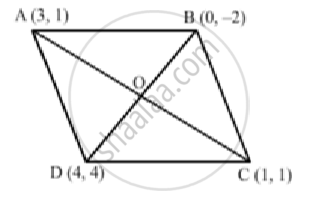# Show that the Points a (3,1) , B (0,-2) , C(1,1) and D (4,4) Are the Vertices of Parallelogram Abcd. - Mathematics

Show that the points A (3,1) , B (0,-2) , C(1,1)  and D (4,4) are the vertices of parallelogram ABCD.

#### Solution

The points are A (3,1) , B (0,-2) , C(1,1)  and D (4,4)

Join AC and BD, intersecting at O.We know that the diagonals of a parallelogram bisect each other ".Midpoint of AC" = ((3+1)/2 , (1+1)/2) = (4/2,2/2) = (2,1)

"Midpoint of BD " ((0+4)/2 , (-2+4)/4) = (4/2,2/2) = (2,1)

Thus, the diagonals AC and BD have the same midpoint

Therefore, ABCD is a parallelogram.

Concept: Area of a Triangle
Is there an error in this question or solution?

#### APPEARS IN

RS Aggarwal Secondary School Class 10 Maths
Chapter 16 Coordinate Geomentry
Q 24